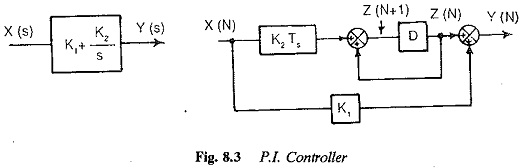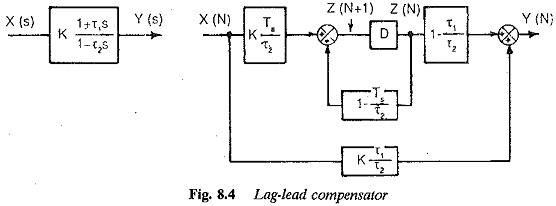## Feedback Control in Drive System:

Feedback Control in Drive System – A closed loop control of a variable speed drive system employing thyristor power converters is normally a discrete time system when a microprocessor is implemented for control purposes The system may be linear or non-linear

The power converter itself is a discrete time system due to switching of the thyristors. Just as a continuous system is represented by differential equations and Laplace transform, a discrete data system can be represented by differ­ence equations and Z-transform. The state space equations are difference equations. The speed loop and current loop have their own time constants and sampling frequencies. The system has multirate sampling. Compared to these the sampling rate of the converter is negligible. The state variable feedback may be employed using the state space techniques which can be ap­plied to both linear and nonlinear systems. The controllers for such feedback control of a variable speed system are shown in Figs 8.3 and 8.4.The microprocessor may be programmed, to implement the necessary con­trollers and limiters. These also have their own sampling rates. The compen­sators in the current and speed loops are introduced to improve the behaviour of the system. These are normally PI, PID, or lag-lead compensators. In the hardware oriented system using discrete analog components, a controller is specified by its transfer function in Laplace transform. In digital control using either digital components or microprocessors, a controller is developed based on its time domain difference equations.

The Laplace transfer function of an analog controller must be suitably transformed to time domain difference equations to develop an equivalent digital controller.

A microprocessor based compensator has the following features:

1. It is cheaper than its analog counterpart.